## Mersenne Number

A number of the form(1)

foran Integer is known as a Mersenne number. The Mersenne numbers are therefore 2-Repdigits, and also the numbers obtained by setting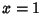in a Fermat Polynomial. The first few are 1, 3, 7, 15, 31, 63, 127, 255, ... (Sloane's A000225).

The number of digits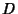in the Mersenne numberis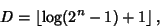(2)

whereis the Floor Function, which, for large, gives(3)

In order for the Mersenne numberto be Prime,must be Prime. This is true since for Compositewith factors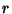and,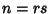. Therefore,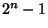can be written as, which is a Binomial Number and can be factored. Since the most interest in Mersenne numbers arises from attempts to factor them, many authors prefer to define a Mersenne number as a number of the above form(4)

but withrestricted to Prime values.

The search for Mersenne Primes is one of the most computationally intensive and actively pursued areas of advanced and distributed computing.

See also Cunningham Number, Eberhart's Conjecture, Fermat Number, Lucas-Lehmer Test, Mersenne Prime, Perfect Number, Repunit, Riesel Number, Sierpinski Number of the Second Kind, Sophie Germain Prime, Superperfect Number, Wieferich Prime

References

Pappas, T. Mersenne's Number.'' The Joy of Mathematics. San Carlos, CA: Wide World Publ./Tetra, p. 211, 1989.

Shanks, D. Solved and Unsolved Problems in Number Theory, 4th ed. New York: Chelsea, pp. 14, 18-19, 22, and 29-30, 1993.

Sloane, N. J. A. Sequence A000225/M2655 in An On-Line Version of the Encyclopedia of Integer Sequences.'' http://www.research.att.com/~njas/sequences/eisonline.html and Sloane, N. J. A. and Plouffe, S. The Encyclopedia of Integer Sequences. San Diego: Academic Press, 1995.